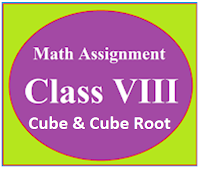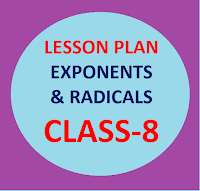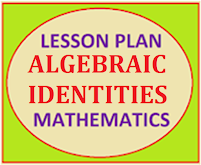## Posts

Showing posts from June, 2023

### Resource Centre Mathematics

Resource Centre Mathematics Mathematics worksheet, mathematics basic points and formulas, mathematics lesson plan, mathematics multiple choice questions Workplace Dashboard CBSE Syllabus For Session 2023-24 For  :   Classes IX & X    |   Classes XI & XII Watch Videos on Maths Solutions CLASS IX MATHEMATICS FORMULAS &  BASIC CONCEPTS

### Math Assignment Class VIII | Cube & Cube Root CH-2MATH ASSIGNMENT CLASS VIII |  CUBE & CUBE ROOT Download or Print, free  assignment with answer key  for   Class  8  Cube & Cube Root .   Important and extra questions that cover all topics of Cube & Cube Root and is useful and helpful for the students. Math Assignment  Class VIII | Cube & Cube Root

### Lesson Plan Math Class 8 | Exponents & Radicals CH-3E- LESSON PLAN   SUBJECT MATHEMATICS    CLASS- 8 Lesson Plan for CBSE mathematics class 8 Exponents & Radicals, Step by step teaching strategy for mathematics teachers. Perfect lesson plan which makes the teaching learning process perfect E-LESSON PLAN MATHEMATICS CLASS-VIII CHAPTER-3 EXPONENTS & REDICALS NAME OF THE TEACHER DINESH KUMAR CLASS VIII CHAPTER 03 SUBJECT MATHEMATICS TOPIC EXPONENTS & RADICALS  DURATION : 8 Class Meetings PRE- REQUISITE KNOWLEDGE:- For batter understanding of this topic students should the following skills Basic arithmetic operations: Familiarity with square numbers: Understanding of square roots: Basic algebraic skills:

### Lesson Plan Math Class 8 | Algebraic Identities CH-7E- LESSON PLAN   SUBJECT MATHEMATICS    CLASS- 8 Lesson Plan for CBSE mathematics class 8 Algebraic Identities, Step by step teaching strategy for mathematics teachers. Perfect lesson plan which makes the teaching learning process perfect E-LESSON PLAN MATHEMATICS CLASS-VIII CHAPTER-7 ALGEBRAIC IDENTITIES NAME OF THE TEACHER DINESH KUMAR CLASS VIII CHAPTER 07 SUBJECT MATHEMATICS TOPIC ALGEBRAIC IDENTITIES  DURATION : 15 Class Meetings PRE- REQUISITE KNOWLEDGE:- Students should have prior knowledge of variables, expressions, and equations. They should be familiar with basic operations such as addition, subtraction, multiplication, and division. Students should have a grasp of exponent notation and the concept of raising a number to a power. They should be familiar with the rules of exponents, such as multiplying and dividing exponents. Students should have experience simplifying algebraic expressions by combining like terms and performing basic operations. They should be able to facto